# Trouble in High-Dimensional Land

How volume breaks down in high dimensions

Let's dive into the world of high-dimensional geometry!

When considering high-dimensional spaces (4 dimensions or higher), we rely on mental models and intuitions from 2D or 3D objects which generalize poorly to high dimensions. This is especially in machine learning, where estimators, decision boundaries, and pretty much everything else as well are defined in $d$-dimensional space (where $d$ is very high), and all our insights often collapse. This post will attempt to highlight some peculiarities of high-dimensional spaces, and their implications for machine learning applications.

## Volumes Concentrate on the Outside¶

In high-dimensional spaces, volume concentrates on the outside, exponentially more so, as dimension increases.

Let's first look at this fact through "hypercubes": when $d=1$, this is an interval, when $d=2$, a square, when $d=3$, a cube, and so on. Mathematically, a hypercube with edge-length $l$ centered at the origin corresponds to the set $$\mathcal{A}_{d}(l) = \{x \in \mathbb{R}^d ~~\vert~~ \|x\|_\infty \leq \frac{l}{2}\}$$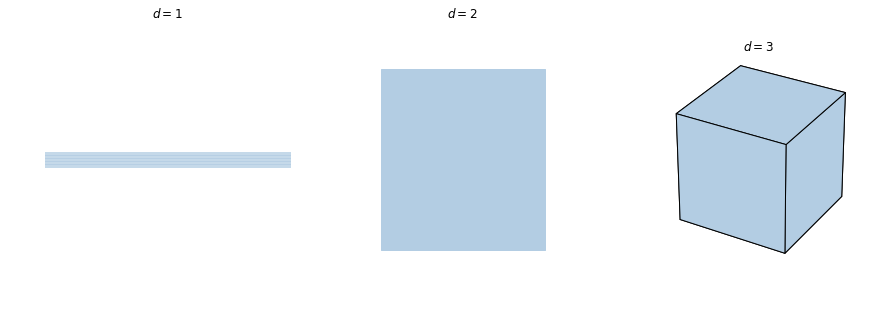Volumes in $\mathbb{R}^d$ are calculated exactly like they are in 2 or 3 dimensions: the volume of a hyper-rectangle is the product of all of the edge lengths.By these calculations, hypercubes $\mathcal{A}_d(l)$ will have volume $\prod_{k=1}^d l = l^d$.

Now, volumes of different dimensional objects aren't directly comparable (it's like comparing apples and oranges), but what we can look at are relative volumes.

Say we have two hypercubes, one of length $l$ and another of $\frac{l}{3}$, what is the relative volume of the smaller cube to the larger cube? How does this proportion change as the dimension increases? Let's first visualize in the dimensions where we can.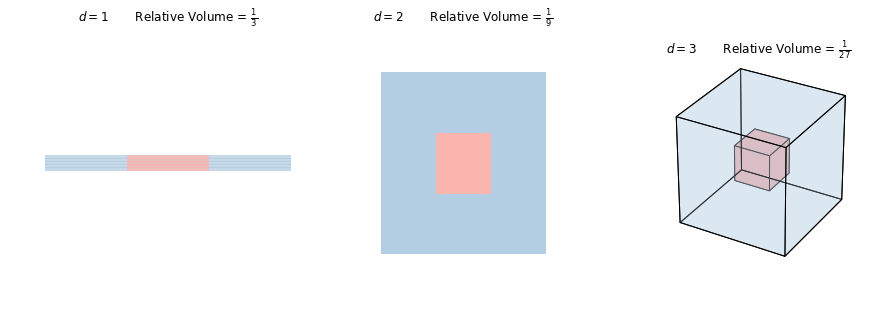Our visualizations indicate that as dimension increases, the relative volume of the smaller cube vanishes exponentially fast. We can confirm this mathematically as well with a simple calculation:

$$\text{Relative Volume} = \frac{\text{Volume}(\mathcal{A}_{d}(\frac{l}{3}))}{\text{Volume}(\mathcal{A}_{d}(l))} = \frac{(l/3)^d}{l^d} = \left(\frac{1}{3}\right)^d$$

This implies that most of the volume in a hypercube lies around the edges (near the surface), and that very little volume lies in the center of the cube.

Why is this an issue for machine learning? Most optimization problems in machine learning can be written of the form:

$$\min_{x \in U_d} ~~~f(x)$$

where $U_d = A_d(1)$ is a unit hypercube. In many applications (including reinforcement learning), the function $f$ is sufficiently complicated that we can only evaluate the value of a function at a point, but no access to gradients or higher-order data from the function. A typical solution is exhaustive search: we test a grid of points in the space, and choose the point that has the best value.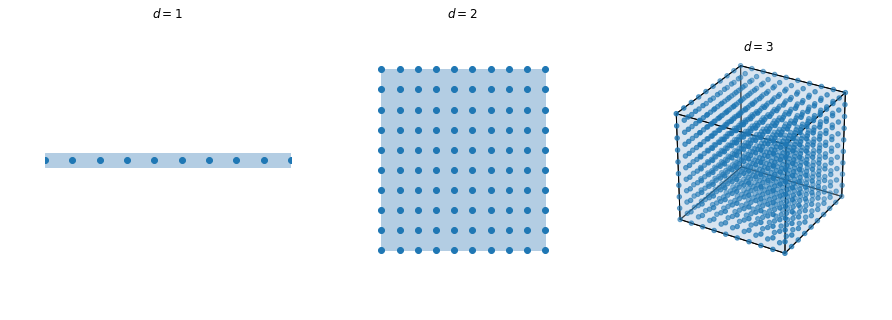The number of points we need to test to get the same accuracy scales exponentially with dimension, for the exact same argument as the volume. To get accuracy $\varepsilon$ (that is $\left|f(\hat{x})-f(x^*)\right| < \varepsilon$ where $\hat{x}$ is our estimate and $x^*$ is the optimal point), the number of points we need to test is on the order of $\left(\frac{1}{\varepsilon}\right)^d$, which is exponential in dimension (a rigorous proof can be given assuming $f$ is Lipschitz continuous). This is often referred to as optimization's curse of dimensionality.

A similar problem exists when computing expectations of functions: a naive way one might compute an expectation is by evaluating the function on a grid of points, and averaging the values like in a Riemannian sum, and computing in this way would also take time exponential in dimension.

## Spheres and their Equators¶

Instead of considering cubes now, let's think about spheres. In particular, we'll think about the unit sphere in $d$ dimensions, which we'll call the $(d-1)$-sphere $S^{(d-1)}$ ($d=2$, a circle, $d=3$, a sphere).

$$S^{(d-1)} = \{x \in \mathbb{R}^d~~\vert~~ \|x\|_2 = 1\}$$

A side note: Calling it a $(d-1)$-sphere may seem odd, but is standard mathematical notation; feel free to mentally substitute $d-1$ with $d$ if it helps improve intuition (the reason it's called a $(d-1)$-sphere is because the sphere is a manifold of dimension $d-1$)

The primary question we'll concern ourselves with is the following:

What proportion of points are near the equator?

We'll approach the problem dually, by asking the question how wide does a band around the equator need to be to capture $1-\varepsilon$ proportion of the points on the sphere?

For the time being, we'll let $\varepsilon = \frac14$ (that is we hope to capture 75% of points), and let's start by investigating $d=2$ (the unit circle)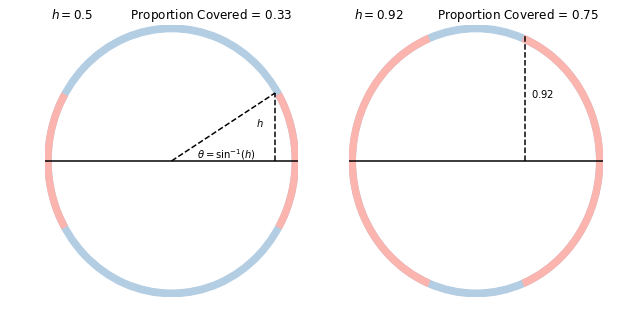For circles ($d=2$), a band of arbitrary height $h$ covers $\frac{4\sin^{-1}(h)}{2\pi} = \frac{2}{\pi}\sin^{-1}(h)$ of the circumference (the picture above serves as a rough proof). To cover 75% of the space, we can solve to find that $h$ needs to be at least $0.92$.

Now let's consider spheres ($d=3$).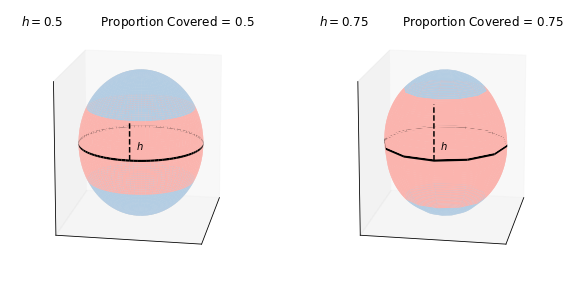For spheres, a band of height $h$ covers a proportion $h$ of the surface area (one can look at spherical caps to derive the formula). Then to cover 75% of the space, we need a band with half-width only $0.75$, which is significantly less than the $0.92$ required for a circle. This seems to indicate the following hypothesis, that we shall now investigate:

Hypothesis: As dimension increases, more of the points on the sphere reside closer to the equator.

Let's jump into $d$ dimensions. For low-dimensional folks like ourselves, analyzing volumes for a $(d-1)$-sphere is difficult, so we'll instead consider the problem probabilistically. What does it mean for a band to cover $1-\varepsilon$ proportion of the sphere? With probability, we can imagine it as saying

If we sample a point uniformly at random from the $(d-1)$-sphere, the probability that it lands in the band is $1-\varepsilon$.

How can we sample a point uniformly at random from the $(d-1)$ sphere? If we recall the symmetry of the multivariate Gaussian distribution about the origin, we encounter an elegant way to sample points from the sphere, by sampling such a vector, and then normalizing it to lie on the sphere.

def sample_sphere(d): # Sample a point uniformly from a (d-1) sphere x = np.random.randn(d) return x / np.linalg.norm(x)

We can investigate this problem empirically by sampling many points from a $(d-1)$-sphere, plot their "x"-coordinates, and find a band that contains 75% of the points. Below, we show it for d = 3 (the sphere), 9, 27, and 81.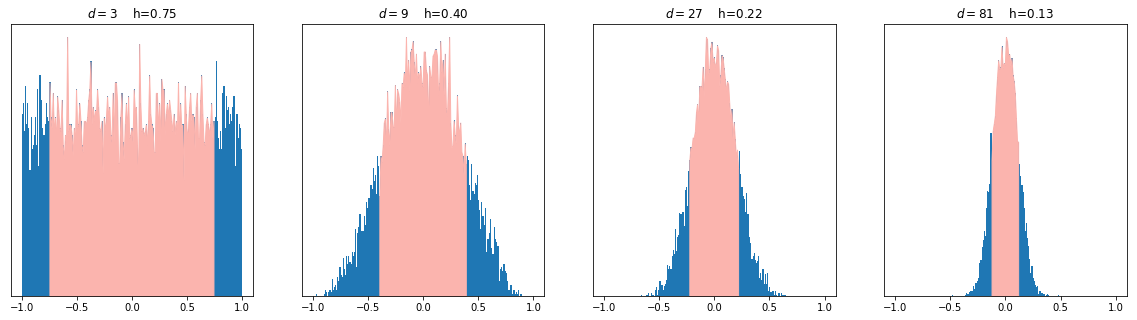Notice that as the dimension increases, the x-coordinates group up very close to the center, and a great majority of them can be captured by very small bands. This yields an interesting point that is not at all intuitive!

In high dimensions, almost all points lie very close to the equator

We can also examine how quickly this clusters by plotting the required height to get 75% of the points as dimension varies: this is shown below.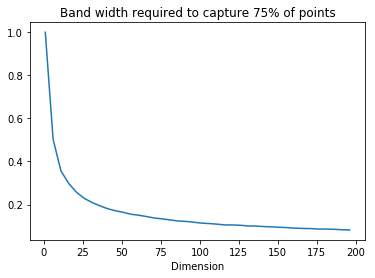We can also prove how quickly points concentrate near the equator mathematically: we show that the square deviation of a point from the equator is distributed according to a Beta($\frac{1}{2}, \frac{d-1}{2}$) distribution, which shows that points concentrate in measure around the equator - that is, the probability that points lie outside of a band of fixed width around the equator goes to $0$ as the dimension increases. See the proof below.

We provide some analysis of this problem.

Consider sampling uniformly on the $(d-1)$-sphere: we can do so by sampling $(Z_1, \dots Z_d) \sim \mathcal{N}(0, I_d)$, and then normalizing to get $(X_1, \dots, X_d) = \frac{1}{\sqrt{\sum Z_k^2}}(Z_1, \dots Z_d)$. What is the distribution of $X_1$? First, let's consider what the distribution of $X_1^2$ is:

$$X_1^2 = \frac{Z_1^2}{\sum Z_k^2} = \frac{Z_1^2}{Z_1^2 + \sum_{k > 1} Z_k^2}$$

Now, recall that $Z_k^2$ is Gamma($r=\frac12, \lambda=\frac12$) and so by the closure of the family of Gamma distributions, $Z_1^2 \sim \text{Gamma}(r=\frac12, \lambda=\frac12)$ and $\sum_{k > 1} Z_k^2 \sim \text{Gamma}(r=\frac{d-1}{2},\lambda=\frac12)$. Gamma distributions possess the interesting property that if $X \sim \text{Gamma}(r_1, \lambda)$ and $Y \sim \text{Gamma}(r_2, \lambda)$, then $\frac{X}{X+Y} \sim \text{Beta}(r_1, r_2)$. Then we simply have that $X_1^2 \sim \text{Beta}(\frac{1}{2}, \frac{d-1}{2})$.

Now, this is a profound fact, and we can get a lot of insight from this formula, but for the time being, we'll use a simple Markov Bound to show that as $d \to \infty$, $X_1$ converges in probability to $0$ (that is that points come very close to the equator). For an arbitrary $\varepsilon$, $$P(|X| > \varepsilon) = P(X^2 > \varepsilon^2) \leq \frac{E(X^2)}{\varepsilon^2} = \frac{1}{d\epsilon^2}$$

This completes the statement.

## Summary and Perspective: Probability Distributions and the "Typical Set"¶

The core tool in statistical inference is the expectation operator: most operations, whether querying the posterior distribution for Bayesian inference or computing confidence intervals for estimators or doing variational inference, etc. The core problem is then to accurately estimate expectations of some function $g$ with respect to some probability distribution $\pi$ where $\pi$ and $g$ are defined on some high-dimensional space ($\mathbb{R}^d$).

$$\mathbb{E}_{X \sim \pi}[g(X)] = \int_{\mathbb{R}^d} g d\pi = \int_{\mathbb{R}^d} g(x) f_\pi(x) dx$$

In the first section, we spent a little time discussing how one may compute this expectation integral: previously, we talked about evaluating the integrand at a grid of points, and averaging (as in a Riemann sum) to arrive at our estimate. However, in practice, we don't need to evaluate at all the points, only at the points that contribute meaningfully to the integral, that is we want to only evaluate in regions of high probability (places where points concentrate).

The previous two sections have hinted at the following fact:

For probability distributions in high-dimensional spaces, most of the probability concentrates in small regions (not necessarily the full space).

• For points sampled at uniform from inside a hypercube, with overwhelming probability, it will be near the surface of the hypercube and not in the center.
• For points sampled at uniform from the surface of a hypersphere, with overwhelming probability, the points will lie near the equator of the sphere.

This concept can be made rigorous with the typical set, a set $A_\epsilon$ such that $P_\pi(X \in A_{\epsilon} > 1 - \epsilon)$. Then, if $g(x)$ is well-behaved enough, we can write

$$\mathbb{E}_{X \sim \pi}[g(X)] = \int_{\mathbb{R}^d} g d\pi = \int_{A_{\epsilon}} g d\pi + \int_{A_{\epsilon}^C} g d\pi \approx \int_{A_{\epsilon}} g d\pi$$

What will help us is that for most distributions, this typical set is actually rather small compared to the full high-dimensional space. In the next article, we'll consider how we can efficiently sample from the typical sets of probability distributions, which will introduce us to topics like Markov Chain Monte Carlo, Metropolis-Hastings, and Hamiltonian Monte Carlo.

Feel free to share!
comments powered by Disqus